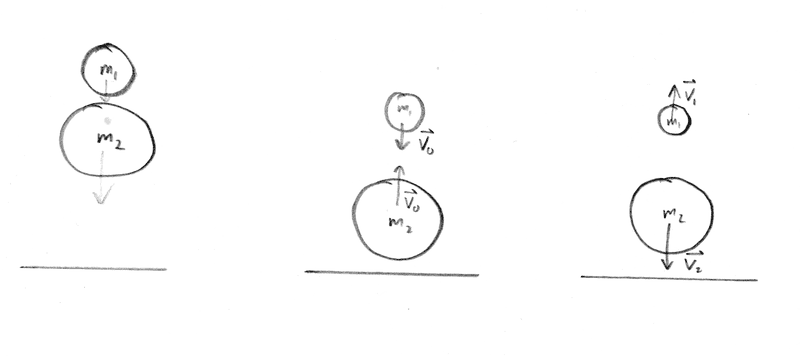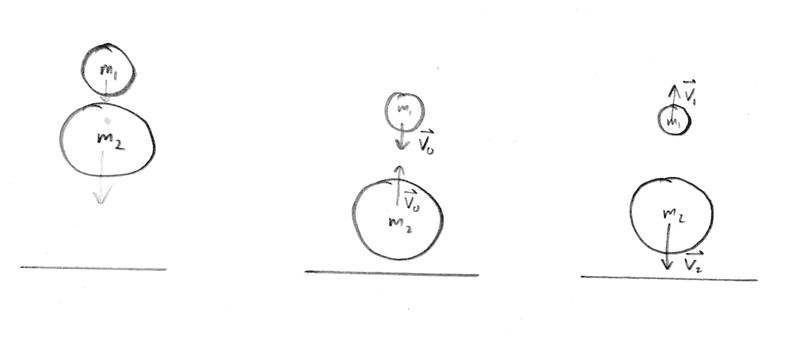# Solving Elastic Collision of Two Balls: Theory & Solutions

• mmfiizik
You should take the derivative of (5) with respect to ##m1/m2## to find the maximum velocity for ##m_1##.f

#### mmfiizik

Homework Statement
Two elastic balls of masses m1 and m2 are placed one above the other (leaving a very small gap between them) and
are released to fall freely on a height from a height h. Assuming that the impact on the floor is elastic, calculate:
a. what must be the ratio m1/m2 of the balls so that the top ball gets the biggest part of total balls energy after impact?
b. What is the maximum height a ball of mass m1 can rise after impact?
h is much bigger than dimension the of balls.
Relevant Equations
Conservation of energy and momentum.With given information in the problem I can't decide which direction balls will go after collision. I assumed they will go in opposite directions. I know that is not a full solution, but I can't come up with anything else.
From conservation of energy we have:
$$m_{2}{v}_{0}^{2}+m_{1}{v}_{0}^{2}=m_{2}{v}_{2}^{2}+m_{1}{v}_{1}^{2} (1)$$
$${v}_{0}=\sqrt{2gh}$$
Conservation of momentum:
$$m_{2}{v}_{0}-m_{1}{v}_{0}=-m_{2}{v}_{2}+m_{1}{v}_{1} (2)$$
from these equations I get:
$$2{v}_{0}={v}_{1}+{v}_{2} (3)$$
$$m_{1}gh+m_{2}gh=\frac{m_{1}{v}_{1}^{2}+m_{2}{v}_{2}^{2}}{2} (4)$$
dividing by m2:
$$2\frac{m_{1}}{m_{2}}gh+2gh={\frac{m_{1}}{m_{2}}{v}_{1}^{2}+{v}_{2}^{2}} (5)$$
Then I replaced v2 from (3), solved quadratic and took derivative with respect to ##m1/m2## , but that gives me absolutely nothing.
I suppose I am making mistake in (2).
I also do not understand derivation of equation of balls speeds in perfectly elastic collision. There is equation:
$$m_{1}{v}_{1}+m_{2}{v}_{2}=m_{1}{v}_{1}^{'}+m_{2}{v}_{2}^{'}$$
And I do not understand how can we take them all positive if we don't know which direction after collision will they go.
If I take all momentum terms positive in equation (2), I get ##v_1=v_2##, which can't be true.

which direction balls will go after collision
Which collision? They start with a small gap, so there will be more than one collision.
And if you are going to use momentum conservation for a collision with the floor you will need to consider the mass of the Earth.

Last edited:
•PeroK and MatinSAR
Homework Statement: Two elastic balls of masses m1 and m2 are placed one above the other (leaving a very small gap between them) and
are released to fall freely on a height from a height h. Assuming that the impact on the floor is elastic, calculate:
a. what must be the ratio m1/m2 of the balls so that the top ball gets the biggest part of total balls energy after impact?
b. What is the maximum height a ball of mass m1 can rise after impact?
h is much bigger than dimension the of balls.
Relevant Equations: Conservation of energy and momentum.

View attachment 327189
With given information in the problem I can't decide which direction balls will go after collision. I assumed they will go in opposite directions. I know that is not a full solution, but I can't come up with anything else.
From conservation of energy we have:
$$m_{2}{v}_{0}^{2}+m_{1}{v}_{0}^{2}=m_{2}{v}_{2}^{2}+m_{1}{v}_{1}^{2} (1)$$
$${v}_{0}=\sqrt{2gh}$$
Conservation of momentum:
$$m_{2}{v}_{0}-m_{1}{v}_{0}=-m_{2}{v}_{2}+m_{1}{v}_{1} (2)$$
from these equations I get:
$$2{v}_{0}={v}_{1}+{v}_{2} (3)$$
$$m_{1}gh+m_{2}gh=\frac{m_{1}{v}_{1}^{2}+m_{2}{v}_{2}^{2}}{2} (4)$$
dividing by m2:
$$2\frac{m_{1}}{m_{2}}gh+2gh={\frac{m_{1}}{m_{2}}{v}_{1}^{2}+{v}_{2}^{2}} (5)$$
Then I replaced v2 from (3), solved quadratic and took derivative with respect to ##m1/m2## , but that gives me absolutely nothing.
I suppose I am making mistake in (2).
I also do not understand derivation of equation of balls speeds in perfectly elastic collision. There is equation:
$$m_{1}{v}_{1}+m_{2}{v}_{2}=m_{1}{v}_{1}^{'}+m_{2}{v}_{2}^{'}$$
And I do not understand how can we take them all positive if we don't know which direction after collision will they go.
If I take all momentum terms positive in equation (2), I get ##v_1=v_2##, which can't be true.
Hello, @mmfiizik ,Below we have your image at full size.The middle figure shows that you are aware that the bottom ball, Ball 2, rebounds (what @haruspex calls the first collision) from the floor with velocity ##v_0##.

Assuming upwards to be positive, the balls then collide with the the initial velocity of Ball 1 being ##-v_0## and the initial velocity of Ball 2 being ##v_0##, where ##v_0=\sqrt{2gh}##.

•MatinSAR and haruspex
Thank you @SammyS for drawing my attention to the diagrams.

With given information in the problem I can't decide which direction balls will go after collision.
You don’t need to care. Just choose which direction to consider positive. The sign of the value that pops out will tell you which way.
Conservation of momentum:
$$m_{2}{v}_{0}-m_{1}{v}_{0}=-m_{2}{v}_{2}+m_{1}{v}_{1} (2)$$
Ok, so you have chosen the convention that ##v_2## is positive down, but all other velocities are positive up. Workable but confusing.
There is equation:
$$m_{1}{v}_{1}+m_{2}{v}_{2}=m_{1}{v}_{1}^{'}+m_{2}{v}_{2}^{'}$$
Correction: that is just the momentum equation again, which you have already used.
I was expecting the equation ##u_1-u_2=u_2'-u_1'##. My comments below are based on that expectation.

That equation can be derived from conservation of energy and momentum. (There is a more general form involving coefficient of restitution when energy is not conserved.)
But in that equation all velocities are positive in the same direction, so to apply it you will need to switch your convention for ##v_2## (##u_2'## above).

Last edited:
•malawi_glenn
But in that equation all velocities are positive in the same direction, so to apply it you will need to switch your convention for ##v_2## (##v_2'## above).
So then (2) should be
$$m_{2}{v}_{0}-m_{1}{v}_{0}=m_{2}{v}_{2}+m_{1}{v}_{1}$$
then I get ##2v_0=v_1-v_2##, which gives me same result if I square it. I think somehow I should find maximum velocity for ##m_1## after first collision with ##m_2##. Then it would get maximum kinetic energy.

So then (2) should be
$$m_{2}{v}_{0}-m_{1}{v}_{0}=m_{2}{v}_{2}+m_{1}{v}_{1}$$
then I get ##2v_0=v_1-v_2##, which gives me same result if I square it. I think somehow I should find maximum velocity for ##m_1## after first collision with ##m_2##. Then it would get maximum kinetic energy.
Just noticed a mistake in my post #4. Please see my corrections.

It would be less confusing to change your convention for ##v_2## to be positive up. Then $$m_{2}{v}_{0}-m_{1}{v}_{0}=m_{2}{v}_{2}+m_{1}{v}_{1}$$
and generically for perfectly elastic collisions ##u_1-u_2=u_2'-u_1'##, where in the present case ##u_1=-v_0, u_2=v_0, u_1'=v_1, u_2'=v_2##.

•malawi_glenn
It would be less confusing to change your convention for ##v_2## to be positive up. Then $$m_{2}{v}_{0}-m_{1}{v}_{0}=m_{2}{v}_{2}+m_{1}{v}_{1}$$
and generically for perfectly elastic collisions ##u_1-u_2=u_2'-u_1'##, where in the present case ##u_1=-v_0, u_2=v_0, u_1'=v_1, u_2'=v_2##.
Ok, so then ##-2v_0=v_2-v_1##
replacing ##v_1## in (2)
$$m_{2}{v}_{0}=m_{2}{v}_{2}+m_{1}{v}_{2}+3m_{1}{v}_{0}$$
$${v}_{2}=\frac{v_{0}(m_{2}-3m_{1})}{m_{1}+m_{2}}$$
Energy converted = first ball energy / total energy
$$E=\frac{m_{2}v_{2}^{2}}{m_{1}v_{0}^{2}+m_{2}v_{0}^{2}}$$
$$E=\frac{m_{2}^{2}+9m_{1}^{2}-6m_{1}m_{2}}{m_{2}^{2}+m_{1}^{2}+2m_{1}m_{2}}\\$$
Let ##k=m1/m2##
$$E=\frac{(1-3k)^2}{(1+k)^2}$$
Maximum energy which first ball gets is at:
$$k=\frac{1}{3}$$
Is it right?

You appear to have minimised the energy of ##m_2##, without saying so?

PS I think it should be ##(1+k)^3## on the denominator.

•MatinSAR
PPS if you look carefully, there is a massive short cut for this question!

Ok, so then ##-2v_0=v_2-v_1##
replacing ##v_1## in (2)
$$m_{2}{v}_{0}=m_{2}{v}_{2}+m_{1}{v}_{2}+3m_{1}{v}_{0}$$
$${v}_{2}=\frac{v_{0}(m_{2}-3m_{1})}{m_{1}+m_{2}}$$
Energy converted = first ball energy / total energy
$$E=\frac{m_{2}v_{2}^{2}}{m_{1}v_{0}^{2}+m_{2}v_{0}^{2}}$$
$$E=\frac{m_{2}^{2}+9m_{1}^{2}-6m_{1}m_{2}}{m_{2}^{2}+m_{1}^{2}+2m_{1}m_{2}}\\$$
Let ##k=m1/m2##
$$E=\frac{(1-3k)^2}{(1+k)^2}$$
Maximum energy which first ball gets is at:
$$k=\frac{1}{3}$$
Is it right?
No.

First, a zero derivative only implies a local extremum. It is not necessarily a local maximum, and even if it is that, not necessarily the absolute maximum.
What do you get for ##v_2## if you plug in k=1/3?

Secondly, you are not asked for the maximum of ##v_2## anyway. You are only asked for conditions on the mass ratio such that ##m_2## gets the lion's share of the energy.

And as @PeroK noted, the denominator should be ##(1+k)^3##.

No.

First, a zero derivative only implies a local extremum. It is not necessarily a local maximum, and even if it is that, not necessarily the absolute maximum.
What do you get for ##v_2## if you plug in k=1/3?

Secondly, you are not asked for the maximum of ##v_2## anyway. You are only asked for conditions on the mass ratio such that ##m_2## gets the lion's share of the energy.

And as @PeroK noted, the denominator should be ##(1+k)^3##.
Yes, I made some mistakes in previous solution.
Energy converted = first ball energy / total energy
$$E=\frac{m_{2}v_{2}^{2}}{m_{1}v_{0}^{2}+m_{2}v_{0}^{2}}$$
$$E=\frac{m_{2}^{2}+9m_{1}^{2}-6m_{1}m_{2}}{m_{2}^{2}+m_{1}^{2}+2m_{1}m_{2}}\\$$

Expression for energy gain should be written for the first ball, not the second.
$$E=\frac{m_{1}v_{1}^{2}}{m_{1}v_{0}^{2}+m_{2}v_{0}^{2}}$$
solving ##v_1## from (2)
$$v_{1}=\frac{v_{0}(3m_{2}-_{}m_{1})}{m_{1}+m_{2}}$$
replacing ##v_1^2## gives:
$$E=\frac{k(3-k)^{2}}{(k+1)^3}$$
Derivative of that expression have zeros at:
$$k=3$$
and
$$k=\frac{1}{3}$$
First, a zero derivative only implies a local extremum. It is not necessarily a local maximum, and even if it is that, not necessarily the absolute maximum.
I don't know how should I prove it for the absolute maximum.
I believe that should imply that maximum energy loss for ##m_1## is when ##m_1=3m_2##, because it just stops ##(v_1=0)## and so all kinetic energy is transferred to ##m_2##.
And minimum energy loss for ##m_1## is when ##3m_1=m_2##, because then ##m_2## ball stops and first ball gets all of the kinetic energy from the second ball. I think I should not take into account potential energy, because gap between them is very small and before collision they are almost on the ground.

Yes, I made some mistakes in previous solution.

Expression for energy gain should be written for the first ball, not the second.
$$E=\frac{m_{1}v_{1}^{2}}{m_{1}v_{0}^{2}+m_{2}v_{0}^{2}}$$
solving ##v_1## from (2)
$$v_{1}=\frac{v_{0}(3m_{2}-_{}m_{1})}{m_{1}+m_{2}}$$
replacing ##v_1^2## gives:
$$E=\frac{k(3-k)^{2}}{(k+1)^3}$$
Derivative of that expression have zeros at:
$$k=3$$
and
$$k=\frac{1}{3}$$

I don't know how should I prove it for the absolute maximum.
I believe that should imply that maximum energy loss for ##m_1## is when ##m_1=3m_2##, because it just stops ##(v_1=0)## and so all kinetic energy is transferred to ##m_2##.
And minimum energy loss for ##m_1## is when ##3m_1=m_2##, because then ##m_2## ball stops and first ball gets all of the kinetic energy from the second ball. I think I should not take into account potential energy, because gap between them is very small and before collision they are almost on the ground.
You do not seem to have understood this comment:
you are not asked for the maximum of v2 anyway. You are only asked for conditions on the mass ratio such that m2 gets the lion's share of the energy
You are still thinking in terms of maximising ##m_2##'s energy, which is not the task.
You are to find those values of the ratio (a range or ranges) such that it has more KE than ##m_1## does after their collision.

•MatinSAR
Actually, the top ball is the ball with mass, ##m_1##.

•haruspex, mmfiizik and hutchphd
You do not seem to have understood this comment:

You are still thinking in terms of maximising ##m_2##'s energy, which is not the task.
You are to find those values of the ratio (a range or ranges) such that it has more KE than ##m_1## does after their collision.
But in the task there is written biggest part of energy, not bigger. So there is only one ratio of masses with which ##m_1##'s KE is biggest, right? And I have to maximize for ##m_1## energy, because it's the top one.

But in the task there is written biggest part of energy, not bigger. So there is only one ratio of masses with which ##m_1##'s KE is biggest, right? And I have to maximize for ##m_1## energy, because it's the top one.
True, it is ambiguous. One way or another it is grammatically inconsistent. Should say either "bigger part" or "biggest amount".
Taking the biggest amount view…
And minimum energy loss for ##m_1## is when ##3m_1=m_2##, because then ##m_2## ball stops and first ball gets all of the kinetic energy from the second ball.
Yes, that is the best approach.

•mmfiizik